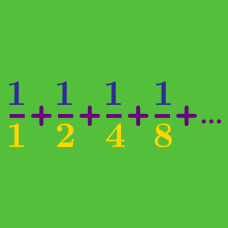Calculus

# Telescoping Series - Sum

Evaluate

$\frac{1}{4\cdot10}+\frac{1}{10\cdot16}+\frac{1}{16\cdot22}+\frac{1}{22\cdot28}+\frac{1}{28\cdot34} .$

$\frac{3}{1 \cdot 2 \cdot 3} + \frac{5}{2 \cdot 3 \cdot 4} + \frac{7}{3 \cdot 4 \cdot 5} + \cdots + \frac{81}{40 \cdot 41 \cdot 42} = ?$

Evaluate

$\frac{1}{\sqrt{4}+\sqrt{7}}+\frac{1}{\sqrt{7}+\sqrt{10}}+\cdots+\frac{1}{\sqrt{397}+\sqrt{400}}.$

Suppose $a,b,c,$ and $d$ are constants such that the following holds for all real numbers $x$ such that all denominators are nonzero: \begin{aligned} & \frac{10}{x(x+10)}+\frac{10}{(x+5)(x+15)}+\frac{10}{(x+10)(x+20)} \\ &+ \frac{10}{(x+15)(x+25)}+\frac{10}{(x+20)(x+30)} \\ &= \frac{a(x^2+30x+75)}{x(x+b)(x+c)(x+d)}. \end{aligned} What is the value of $a+b+c+d?$

If $f(x)=4x^2-1,$ what is the value of

$\frac{1}{f(1)}+\frac{1}{f(2)}+\frac{1}{f(3)}+\cdots+\frac{1}{f(84)}?$

×

Problem Loading...

Note Loading...

Set Loading...